# Texas Go Math Grade 3 Lesson 2.1 Answer Key Equal Shares

Refer to our Texas Go Math Grade 3 Answer Key Pdf to score good marks in the exams. Test yourself by practicing the problems from Texas Go Math Grade 3 Lesson 2.1 Answer Key Equal Shares.

## Texas Go Math Grade 3 Lesson 2.1 Answer Key Equal Shares

Essential Question
Why do you need to know how to make equal shores?

Unlock the Problem

Four friends share 2 small pizzas equally. What are two ways the pizza could be divided equally? How much pizza will each friend get?

• How might the two ways be different?
The ways depend on how big the slices we want. We need to cut each pizza into 2 or 4 or 6 or 8 etc. equal pieces.

A whole is all of the parts of one shape or group. Equal parts are exactly the same size.

Draw to model the problem.

Draw 2 circles to show the pizzas.

One Way
There are ___ friends.
Cut each pizza into 4 slices.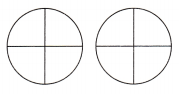There are ___ equal parts in each pizza.
Each friend can have 2 equal parts. Each friend will get 2 fourths of a pizza.
There are 4 friends.
There are 8 equal parts in each pizza.

Explanation:
There are 4 friends. So we cut each pizza into 4 slices.
There are 8 equal parts in each pizza.
Each friend can have 2 equal parts. So each friend will get 2 fourths of a pizza.

Another Way
There are __ friends.
Cut each pizza into 2 slices.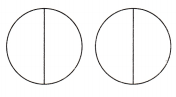There are __ equal parts in each pizza.
Each friend can have 1 equal part. Each friend will get 1 half of a pizza.
There are four friends.

Explanation:
There are 4 friends. So cut the pizza into 2 slices making 4 slices altogether. And each friend gets 1 equal part. So each friend gets 1 half of a pizza.

• Explain why both ways let the friends have an equal share.
The first way is to cut the pizzas into 4 equal slices, making 8 total (4+4). So each person could have 2 equal slices, and make up the 8 equal slices.
The second way is to cut the pizzas into 2 equal slices, making 4 total (2+2). So each person could have 1 equal slice each, making up the 4 slices.

Math Talk
Mathematical Processes
Explain another way the cookies could have been divided. Tell how much each friend will get.

Share and Show

Question 1.
Three friends share 2 cookies equally. Use the picture to find how much each friend gets.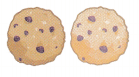Think: There are more friends than cookies.

Draw lines to show how much each person gets. Write the answer.

Texas Go Math Grade 3 Answer Key Pdf Lesson 2.1 Question 2.
8 sisters share 3 brownies equally.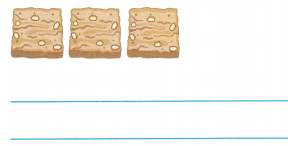$$\frac{3}{8}$$

Explanation:
The 3 brownies are shared among 8 sisters equally. So each sister gets $$\frac{3}{8}$$ of the brownie.

Question 3.
6 neighbors share 4 pies equally.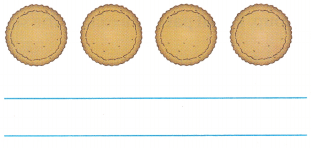$$\frac{4}{6}$$

Explanation:
The 4 pies are equally shared among the 6 neighbors. Therefore each neighbor gets $$\frac{4}{6}$$ of the pie.

Problem Solving

Draw to show how much each person gets. Shade the amount that one person gets. Write the answer.

Question 4.
8 friends share 4 sheets of construction paper equally.
$$\frac{4}{8}$$

Question 5.
6 students share 5 small pizzas equally.
$$\frac{5}{6}$$

Unlock the Problem

Question 6.
H.O.T. Multi-Step Julia has 4 adults and 3 children coming over for dessert. She is going to serve 2 small blueberry pies. If she plans to give each person, including herself, an equal share of pie, how much pie will each person get?a. What do you need to find?
We need to find how much pie will each person get.

b. How will you use what you know about drawing equal shares to solve the problem?
There are a total of 7 people coming over for dessert. There she is going to serve 2 small blueberry pies. By using these we can draw equal shares to solve our problem.

c. Draw a quick picture to find the share of pie each person will get.

d. Complete the sentences.
Julia has ___ adults and ___ children coming over.
In all, there will be ___ people having pie.
She is going to serve ___ blueberry pies.
So, each person will get __________ of a blueberry pie.
Julia has 4 adults and 3 children coming over.
In all, there will be 7 people having pie.
She is going to serve 2 blueberry pies.
So, each person will get $$\frac{2}{7}$$ of a blueberry pie.

Go Math Answer Key Grade 3 Equal Shares of a Shape Question 7.
H.O.T. Multi-Step Keith baked 3 cherry pies and 2 apple pies. He wants to share each type of pie equally among 6 of his neighbors. How much of each type of pie will each neighbor get?$$\frac{3}{8}$$

Explanation:
Keith baked 3 cherry pies and 2 apple pies. A total of 5 pies are baked and will be shared among 6 of his neighbors. So each neighbor gets $$\frac{3}{8}$$ type of pie.

Fill in the bubble for the correct answer choice.

Question 8.
Apply Jake and his three friends bought two apples to share. They divided the apples equally. How much of an apple did Jake and his friends each get?
(A) 1 fourth
(B) 3 fourths
(C) 2 fourths
(D) 2 halves
2 fourths

Explanation:
There is a total of 4 friends and they bought two apples to share. They share the apples equally. Each friend gets 2 fourths of an apple.

Question 9.
Representations Mrs. Post has three children and two cheese sticks. How should she divide the cheese sticks so that each child gets an equal share?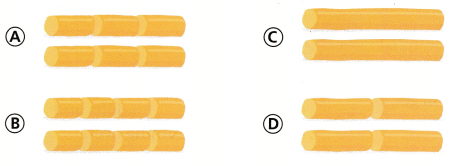option D

Explanation:
Mrs. Post has 3 children and each child gets two cheese sticks. She divided the cheese sticks so that each child gets an equal share of Option D.

Question 10.
Multi-Step There are two adults and six children in Mia’s family. They bought three large sandwiches and cut each
sandwich into equal slices. 1f each person gets an equal share, how much of a sandwich will each person get?
(A) 1 eighth
(B) 3 eighths
(C) 2 eighths
(D) 2 fourths
3 eighths

Explanation:
In the given question there are 2 adults and six children. A total of 8 people are there in Mia’s family. They bought three large sandwiches. If each person has an equal share. So each person gets $$\frac{3}{8}$$ of sandwich share.

Texas Test Prep

Question 11.
Six friends share 2 pizzas equally. How much of a pizza does each friend get?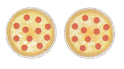(A) 2 sixths
(B) 6 thirds
(C) 6 halves
(D) 3 sixths
2 sixths

Explanation:
Two pizzas are shared with six friends equally. Therefore each friend gets $$\frac{2}{6}$$ of a pizza.

### Texas Go Math Grade 3 Lesson 2.1 Homework and Practice Answer Key

Draw to show how much each person gets. Shade the amount that each person gets. Write the answer.

Question 1.
6 friends share 3 small pies equally.
$$\frac{3}{6}$$

Explanation:
The 3 small pies are shared among 6 friends equally. So each person gets $$\frac{3}{6}$$ of the pie.

Question 2.
8 students share 5 square pieces of cake equally.
$$\frac{5}{8}$$

Explanation:
The 5 square pieces of cake are shared among 8 students equally. So each person gets $$\frac{5}{8}$$ of the cake.

Problem Solving

Question 3.
4 boys share 3 granola bars equally. Use the picture to find how much each boy gets.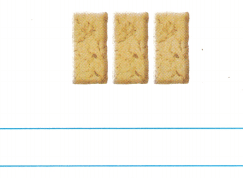$$\frac{3}{4}$$

Explanation:
Here we are trying to share out 3 bars between 4 boys.
We need to divide: 3÷4 and Write the division in the fraction format $$\frac{3}{4}$$.
Each boy will get “three-quarters” of a bar.

Texas Go Math Grade 3 Equal Shares Lesson 2.1 Answer Key Question 4.
Sean makes 2 pizzas. He gives 8 people an equal share of the pizza. How much pizza does each person get?$$\frac{1}{4}$$

Explanation:
Sean made 2 pizzas and gave 8 people an equal share of the pizza. The pizza does each person get is $$\frac{1}{4}$$.

Texas Test Prep

Lesson Check

Question 5.
Jade and five friends had 3 oranges. They shared the oranges equally. How much of an orange did Jade and her friends each get?
(A) 1 fourth
(B) 1 half
(C) 1 third
(D) 2 halves
1 half

Explanation:
Jade and her friends got a half orange each.
Jade and five friends had 3 oranges and they shared the oranges equally. Each of them would get half orange each.

Question 6.
Mr. Mott has two cheese sticks and four children. How should he cut the cheese sticks so that each child gets an equal share?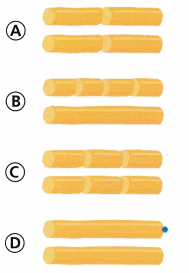Option A

Explanation:
He should cut the cheese sticks as $$\frac{4}{6}$$ so that each child gets an equal share.

Multi-Step Four girls and two boys are working on an art project. They have four square pieces of felt. If each person gets an equal share of the felt, how much of a piece of felt will each person get?
(A) 1 sixth
(B) 1 fourth
(C) 2 fourths
(D) 4 sixths
4 sixths

Explanation:
Four girls and two boys are working on an art project. In total, we have 6 people working on a project. Each person gets an equal share. Each person gets $$\frac{4}{6}$$.

Question 8.
Multi-Step Matt has 3 cherry pies and 2 apple pies. He wants to share each type of pie equally among 4 different families. How much of each type of pie will each family get?(A) 1 half of a cherry pie and 3 fourths of an apple pie
(B) 3 fourths of a cherry pie and 1 half of an apple pie
(C) 3 fourths of a cherry pie and 3 fourths of an apple pie
(D) 3 fourths of a cherry pie and 1 fourth of an apple pie
We need to divide the pies equally among the four families then each family gets $$\frac{1}{2}$$ of the Apple pies and $$\frac{1}{2}$$+$$\frac{1}{4}$$ of the cherry pies.